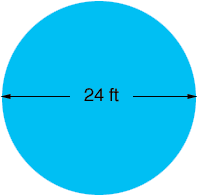Quandaries and Queries My question is: I need to have dirt hauled away from my built in pool and the haulers are asking me how many yards of dirt there is. How would I figure that out? It is a 24 ft round pool and 3 inches of soil depth was dug out. Thank you! Debra Hi Debra, By a "24 ft round pool" I am assuming that you mean 24 feet in diameter.When contractors say "yards" in the context they mean "cubic yards". You have a very short circular cylinder of soil to remove which has a radius of 12 ft and a height of 3 inches. The volume of a circular cylinder is given by volume =r2 h where r is the radius, h is the height andis approximately 3.1416. You want the volume in cubic yards so you should first change all the dimensions to yards. 12 feet is 12/3 = 4 yards 3 inches is 3/12 = 1/4 feet which is 1/41/3 = 1/12 yards. Thus the volume of dirt is421/12 = 4.2 cubic yards Penny Go to Math Central